## Earth Albedo

### Introduction

Earth albedo is the sunlight reflected off the Earth's surface. The Earth albedo induces power in solar cells just like direct sunlight. The Earth albedo model presented here, calculates the amount of Earth albedo arriving at an object in space, maintaining information of direction of the Earth albedo, useful for objects in low Earth-orbit.

The directional information is maintained by partitioning the Earth surface in small cells, and calculating the Earth albedo contribution of each cell, rather than assuming a total amount of albedo from the nadir direction. The partitioning is illustrated in Figure 1.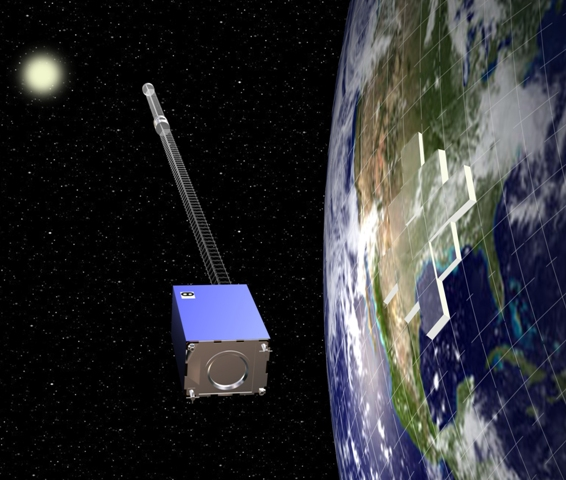Figure 1. Illustration of Earth albedo modeling principle.
(full size)

### Input Data

In order to calculate the Earth albedo, the model needs three input parameters in the ECEF frame: satellite position vector, Sun position vector, Earth surface reflectivity.

The partitioning is defined by the resolution of the input reflectivity data. Reflectivity data is available from the TOMS project. Matlab converted data and pre-processed data (annual means, standard deviations etc.) are available within the Earth Albedo Toolbox, which also includes Matlab functions for loading TOMS data into Matlab manually. The resolution of the TOMS data is 180 x 288 data points. That is 51,840 cells of 1 deg latitude and 1.25 deg longitude. This resolution may be decreased in the toolbox software. Figure 2 shows the plot of the 2001 annual mean of the TOMS reflectivity data.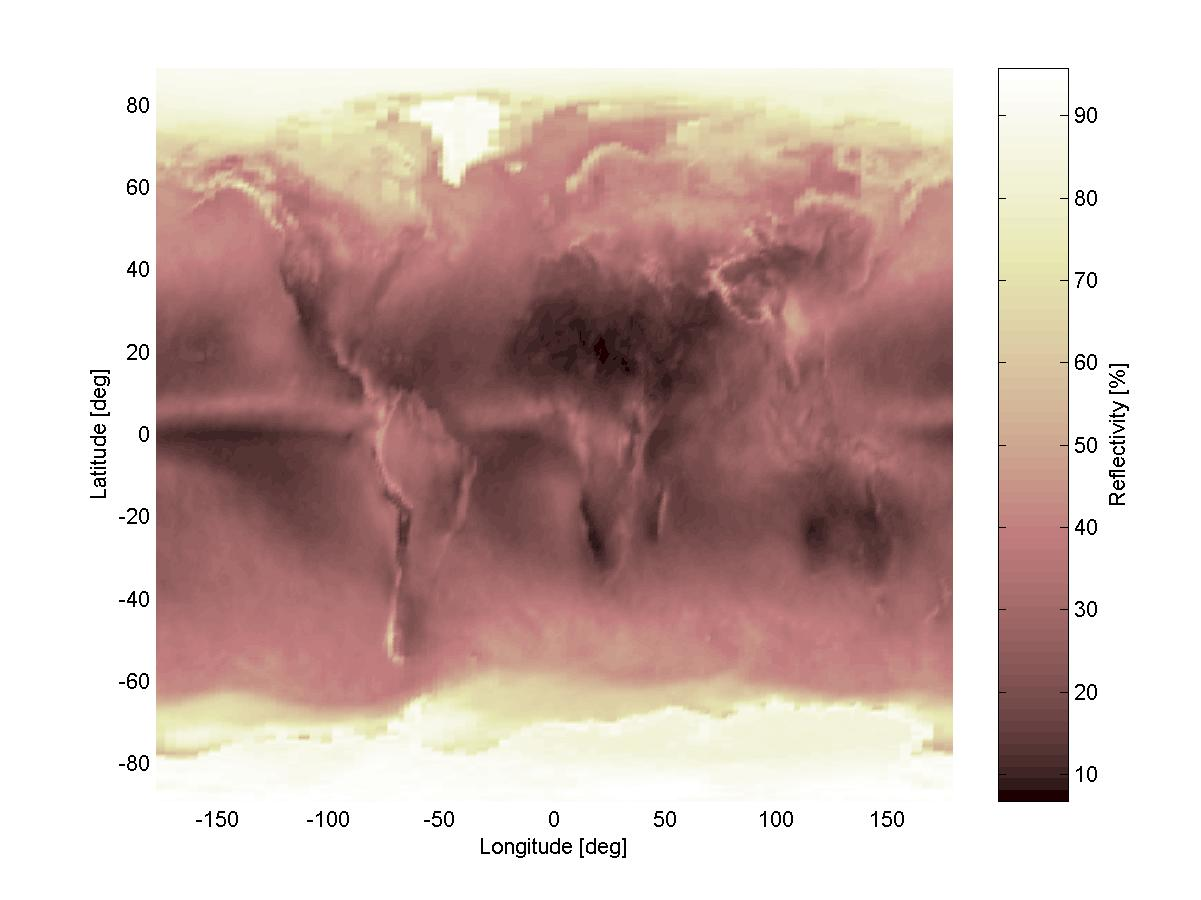Figure 2. Plot of the 2001 annual mean of the TOMS reflectivity data.
(full size)

### Mathematical Model

The model calculates the Earth albedo from each data point in the reflectivity data. The simplified reflection model is shown in Figure 3. The grid point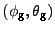defines the longitude and latitude of the data point. This point defines a cell with an area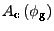expanded by 1 deg latitude and 1.25 deg longitude. The incident irradiance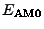arrives from the direction to the Sun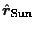, the angle of which is defined from the cell normal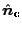. The incident irradiance is assumed to be constant for all cells. The direction of the satellite is defined by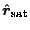, also defined by an angle to the cell normal. The reflected irradiance at satellite distance from the cell center is denoted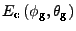.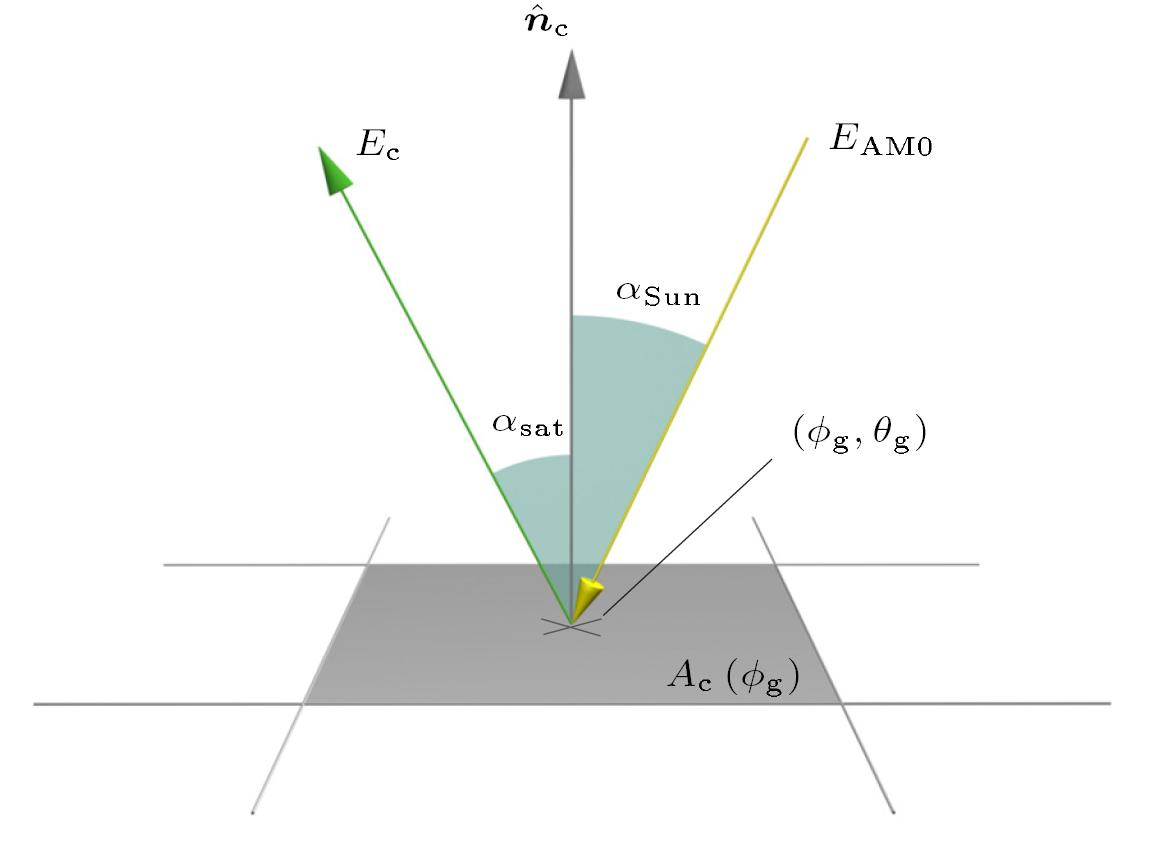Figure 3. Reflection model of s single reflectivity data point.
(full size)

Given the above definitions, a matrix of contributions from each cell in the input data, is calculated by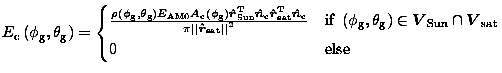,

where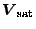is the set of all data points visible from the satellite, and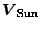is the set of all sunlit data points (or visible from the Sun). The total albedo can be calculated by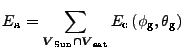.

However, unlike the incident irradiance, the this irradiance is not single directional. When calculating the output of photo voltaics, higher accuracy is achieved by calculating the contribution to the power output from each cell, and incorporating the direction to each cell in the calculations. This may be done using the following equation, assuming simple cosine dependency on output current current and angle of incidence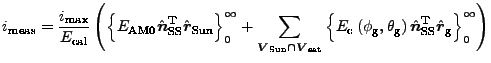,

where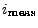is the measured output on the photo voltaics,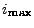is the output current measured, when illuminated with an irradiance of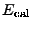, and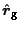is the direction to the grid point (or cell center). The angle of incidence is measured relative to the normal to the photo voltaic plane,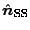, in this case a Sun sensor.

### Application

By applying the annual mean reflectivity data of 2001, the Earth albedo may be calculated given any satellite/Sun constellation described in the Earth Centered Earth Fixed frame. It should be noted that applying annual means as opposed to daily reflectivity data has shown to have very small impact on the resulting Sun sensor currents, hence the mean data is typically applied.

#### Single Calculation

The annual mean reflectivity data of 2001 are plotted in Figure 4, where the sunlit set of data points is shown. The spherical coordinates of the Sun are (NB: the function albedo.m takes the Cartesion ECEF coordinates as parameter)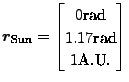.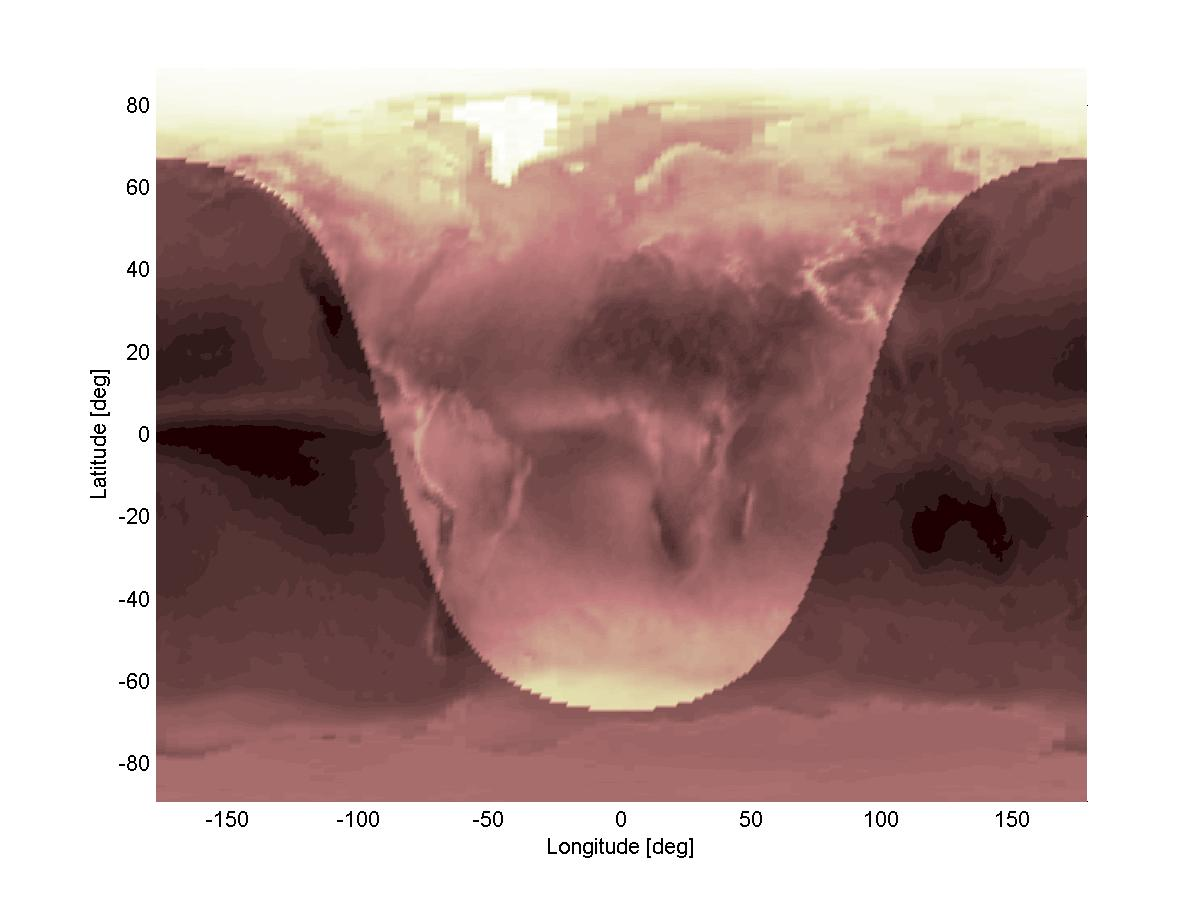Figure 4. Annual mean reflectivity data of 2001. The sunlit data points are emphasized.
(full size)

The satellite is placed at an altitude of 800km. The field of view of the satellite is illustrated in Figure 5. The spherical coordinates of the satellites are (NB: the function albedo.m takes the Cartesion ECEF coordinates as parameter)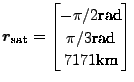.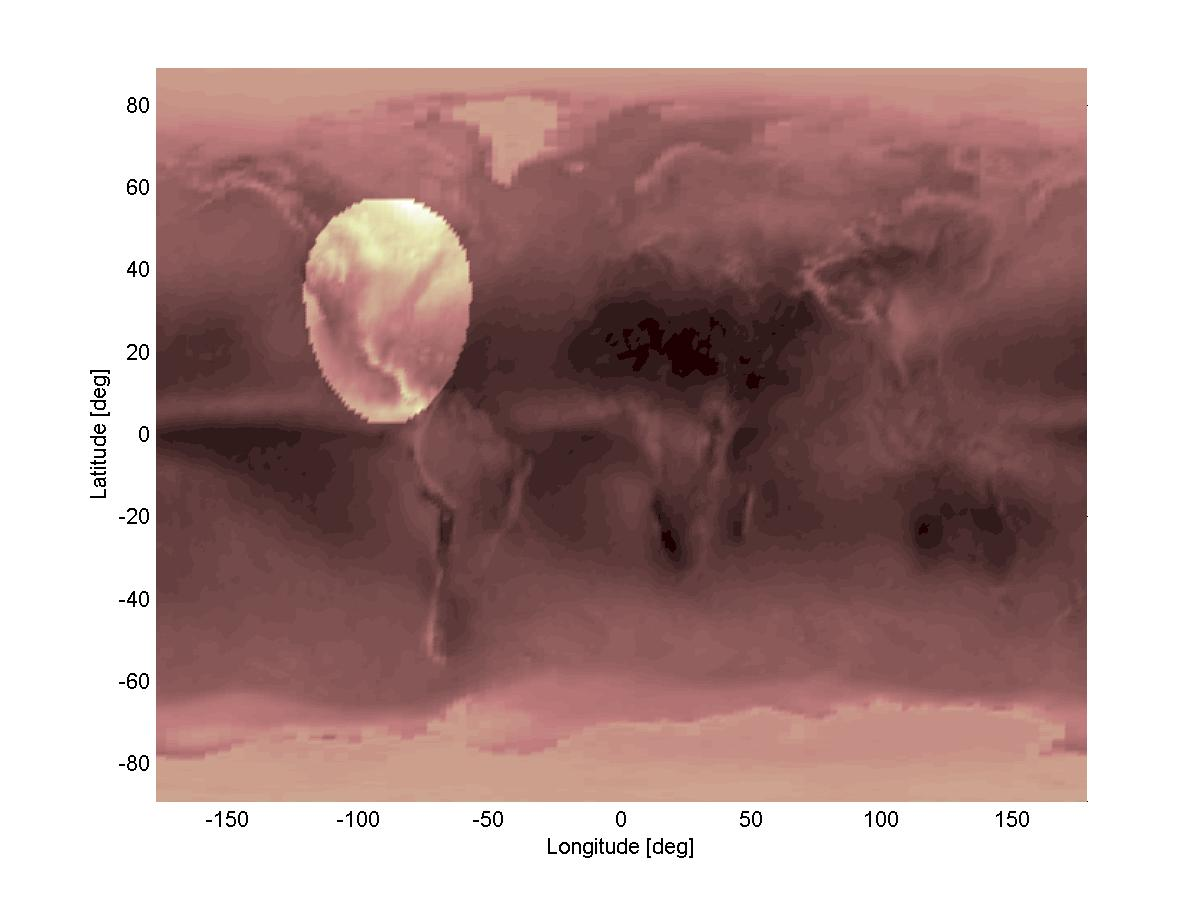Figure 5. Annual mean reflectivity data of 2001. The satellite field of view is emphasized.
(full size)

The output of the Earth albedo model is a matrix of Earth albedos from each cell in the input reflectivity data. This matrix is plotted in Figure 6. The total Earth albedo is found by summing up the entire matrix, which yields an irradiance of 74.5 W/m2. Since an AM0 irradiance of 1367 W/m2 was used, the Earth albedo is 5.45%.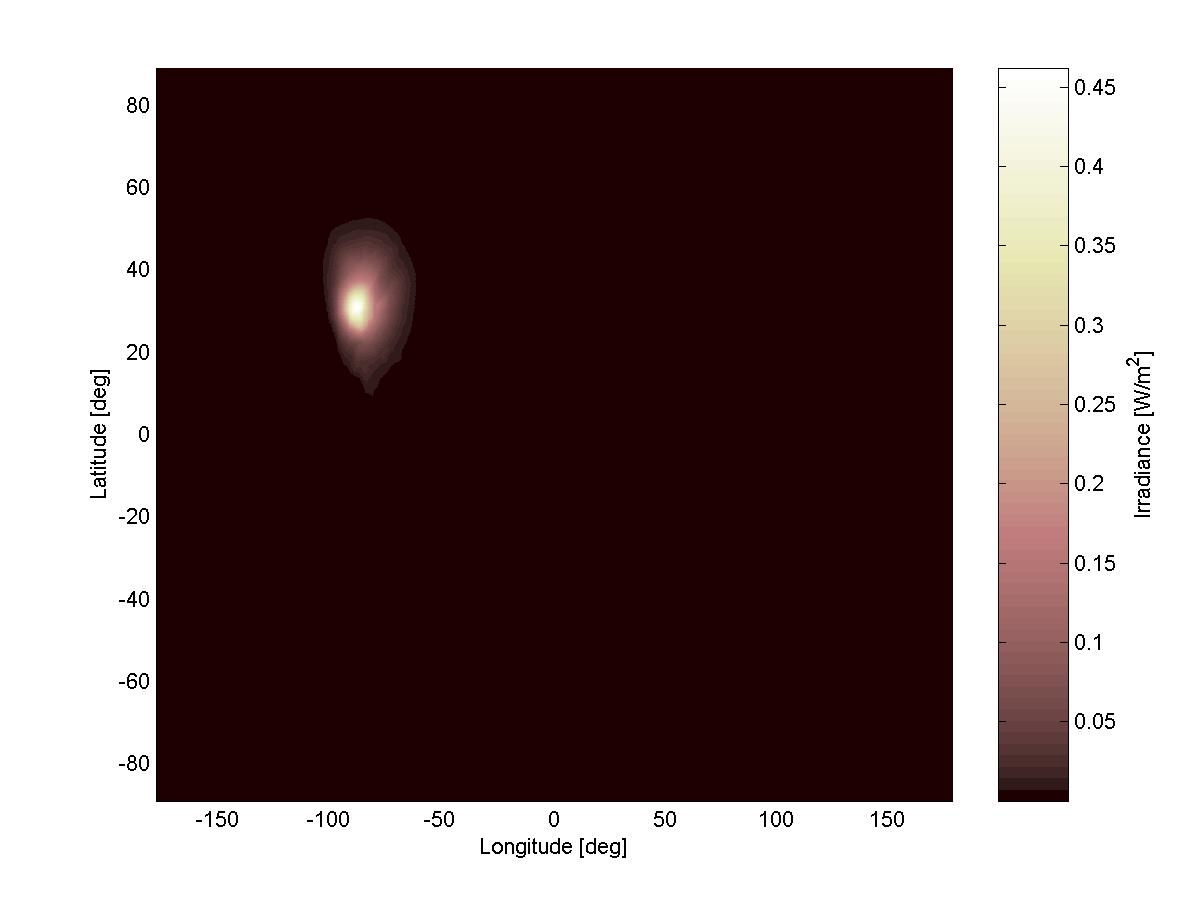Figure 6. Earth albedo model output matrix.
(full size)

#### Simulation

Using the Simulink interface, the Earth albedo can be included in simulations of satellite attitude and orbit. Figure 7 shows the sample points of a simulated orbit of the Ørsted satellite on the 21st of May 2001 from 15:52 to 22:10. The Ørsted satellite is in a low Earth sun synchronous polar orbit, with an altitude between 650km and 850km and 96.1 deg inclination. The orbit period is 100 minutes.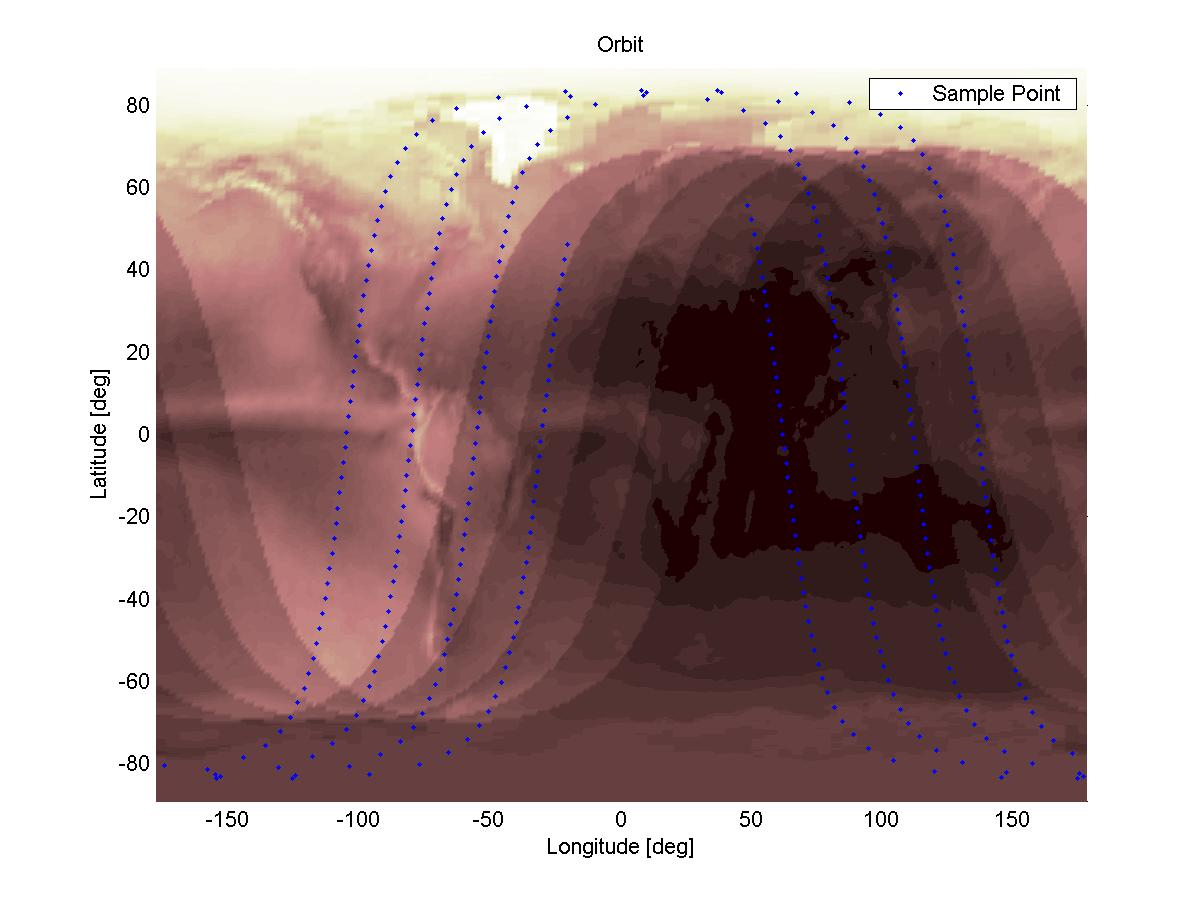Figure 7. Simulated Ørsted orbit on the 21st of May 2001.
(full size)

Figure 7 shows the Ørsted orbit as a series of sample points at which the Earth albedo has been calculated. The resulting total Earth albedo is plotted in Figure 8. The total Earth albedo is given as a percentage of the AM0 irradiance, i.e. 1367W/m2. It is seen from the Figure 7 that the total Earth albedo ranges from 0% in eclipse to almost 40% at maximum.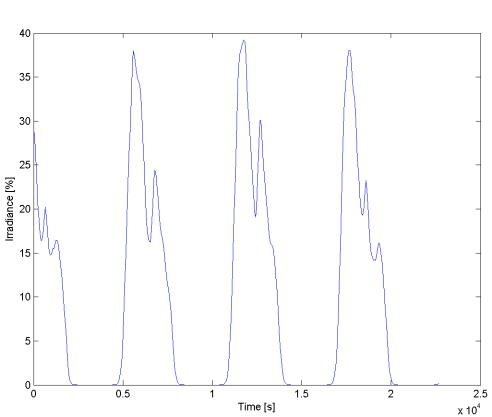Figure 8. Calculated total Earth albedo at the Ørsted satellite on 21st of May 2001 from 15:52 to 22:10.

Figure 8 only shows the total Earth albedo, i.e. the sum over all values in the albedo matrix. The full albedo matrix may be utilized to accurately model the output current on the Sun sensors. The output of the Sun sensor model may be compared to the Ørsted telemetry data for verification of the Earth albedo model and the application in photo voltaic models.

The Sun sensor output model is calibrated by comparing the model output disregarding the Earth albedo contribution to the output current with the Ørsted telemetry. The calibration simply adjusts the solar cell efficiency, since these are known to decay during mission life. The Ørsted satellite is equipped with eight Sun sensors SS1-SS8. The simulated and measured currents from the Sun sensors are shown in Figure 9 after the Sun sensor models have been calibrated. The telemetry is from the same orbit as in the above simulation run, i.e. May 21st 2001 from 15:52 to 22:10. It is seen that the simulated currents in some instances are lower than the measured currents. This is due to the Earth albedo. It should be noted that SS7 has been identified as faulty due to an expected crack in the cover glass of the sensor. Hence no conclusions are based on this sensor. The deviation in SS1 is due to shading from the boom, which is not included in the model.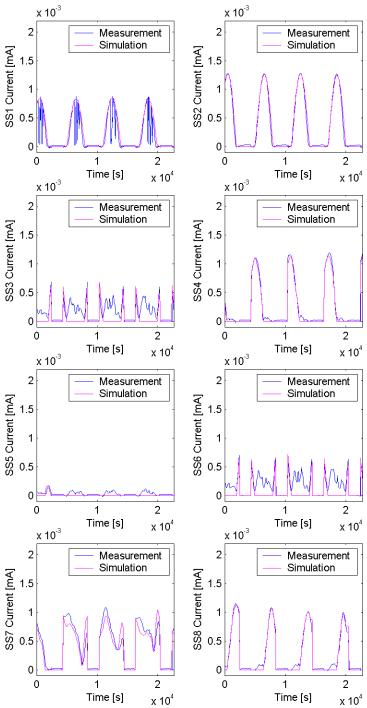Figure 9. Comparison of Sun sensor telemetry and Sun sensor models disregarding the effects of Earth albedo. This comparison has been used to calibrate the Sun sensor models, and the resulting current outputs are shown in the figure.

Being gravity gradient stabilized, due to its 8m boom, the Ørsted satellite is Earth pointing, with the boom pointing away from Earth at all times. This means that Sun sensors SS1 and SS2 are always looking away from Earth and are not expected to show any Earth albedo induced currents. SS3 and SS6 look in the opposite direction and the effects of Earth albedo are most visible on these, as it is also seen in Figure 9. The Earth albedo model output matrix is included in the modeling of the Sun sensor output. The directional information, which is contained by the indexing of the albedo matrix, is applied to calculate the Earth albedo induced currents from each cell on the Earth surface, sunlit and visible from the satellite, at each sample point. The resulting currents are compared to the Ørsted telemetry is shown in Figure 10. It is clear from the figure that the Earth albedo model and Sun sensor output models produce significantly better results. It is also seen that applying the directional information in albedo matrix, the Earth albedo induced currents can be calculated even on Sun sensors which have a normal perpendicular on the Nadir vector (SS4, SS5, SS7, SS8).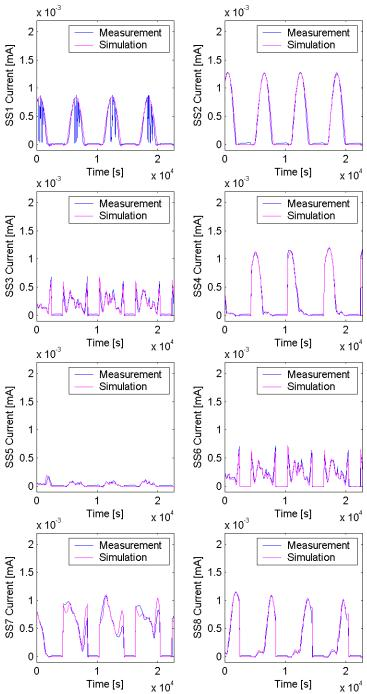Figure 9. Comparison of Sun sensor telemetry and Sun sensor models when including the effects of Earth albedo.